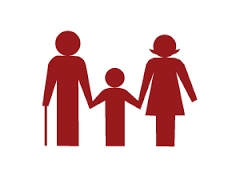# Age question

Jano is three times older than Zdeno. Zdeno is 12 years younger than Janko. How old is Jano, and how old is Zdeno?

j =  18
z =  6

### Step-by-step explanation:

j=3z
z = j-12

j=3·z
z = j-12

j-3z = 0
j-z = 12

Row 2 - Row 1 → Row 2
j-3z = 0
2z = 12

z = 12/2 = 6
j = 0+3z = 0+3 · 6 = 18

j = 18
z = 6

Our linear equations calculator calculates it.Did you find an error or inaccuracy? Feel free to write us. Thank you!

Tips for related online calculators
Do you have a linear equation or system of equations and looking for its solution? Or do you have a quadratic equation?
Do you want to convert time units like minutes to seconds?